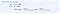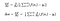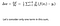# Mathematics behind the parameters update rule:

This article covers the content discussed in the Sigmoid Neuron module of the Deep Learning course and all the images are taken from the same module.

In this article, we discuss the mathematics behind the parameters update rule.

Our goal is to find an algorithm which at any timestamp, tells us how to change the value of w such that the loss that we compute at the new value is less than the loss that we have at the current value.

And if we keep doing this at every step, the loss is bound to decrease no matter where we start from and eventually reach its minimum value.

And Taylor series tells us that if we have a function and if we know its value at a certain point(x in the below case), then its value at a new point which is very close to x can be given by the below expression

And we can see that the Taylor series relates the function value at a new point (x + δx)with the function value at the current point(x)

In fact, the value at the new point is equal to the value at the current point plus some additional terms all of which depends on δx

Now if this δx is such that the quantity which is getting added to f(x)(in brackets in the below image) is actually negative, then we can sure that the function value at a new point is less than the function value at the current point.

So, we need to find δx in such a way so that the quantity in brackets in the above image is negative. The more negative the better because the loss would decrease by more.

The quantity in blue in the above image is the first-order derivative of x.

The quantity in green in the above image is the second-order derivative of x.

The quantity in yellow in the above image is the third-order derivative of x.

If we have f(x) as x³, then all of these quantities would be:

Now we can write the Taylor series for the Loss function as:

The idea is to find the value of δw in such a way that the quantity in the brackets in the below image is negative, then we know that the new loss value would be smaller than the old loss

And since loss depends on b as well, so we want the new loss value to be less than the current loss value for new value of b as well

If we change w or b, the predicted output would change.

If the predicted output changes, then the difference between the predicted output and the true output changes and if that is going to change, the loss value is going to change.

The Taylor series for a vector looks like:

The quantity in brackets in the above image depends on the change in the parameter value. So, we need to find this change vector(δθ or u) such that the quantity in the brackets turns out to be negative and if that happens, we would be sure that the loss would decrease.

Now we can get rid of some of the terms in brackets in the below equation:

Eta is very small, in practice, it would be something around 0.0001 or of that order, if eta is small then the higher powers of eta are going to be even smaller, so even without knowing the exact value of the quantity in yellow in the below image, we can be very sure that this entire term is going to be very small and similarly as we go ahead in the equation where we have eta³ and eta⁴, those terms would even be smaller

So, in practice, all the terms that come later on(in brackets in the below image), are going to be very small and we can get rid of them.

And we can write the equation as an approximation as the below:

And the quantity in yellow in the above image is the first-order partial derivative of loss with respect to theta and the way to compute the partial derivative is that we assume the other variable to act as a constant for example: if the loss depends on w and b and we are computing the partial derivative with respect to w, then we can treat b as a constant.

If our function is below:

then we can compute the partial derivative with respect to ‘w’ as

and this would result in the below assuming b to be constant as we are computing partial derivative:

And similarly, the partial derivative with respect to b would be:

And if we put the above two partial derivatives(with respect to w and b) in a vector, we get a gradient:

And we denote the gradient as:

And for the above case, we can write as:

which means it is the gradient of the function f(θ) with respect to θ and θ actually is just a vector of w and b.

So, going back to our original equation, we have:

The quantity on the left-hand side is the loss value at the new point and is going to a Real no., the current loss value is also a real no., eta is also a small real number, and the other two terms are vector and we are taking their dot product which gives us a real number, so overall on both sides of the equality we have the real numbers.

We can re-write the above equation as:

The quantity in the above image is a dot product between two vectors.

We want the below quantity to be less than 0 and since it is the dot product of two vectors, we want the angle between these two vectors to be greater than 90 but less than equal to 180:Minus(-) sign is there because we have to go in the opposite direction of the gradient.

## Computing Partial Derivatives:

The general recipe that we have is:

So, we have 5 data points as the input, we have chosen the model to be Sigmoid function

And we compute the loss value as:

We can compute the δw as:Consider the square in this equation as the loss function we are using is squared error loss.

The derivative of a sum of quantities is equal to the sum of the derivative of individual quantities.

And now out of the 5 terms in the derivative, let’s consider one term and we will compute its partial derivative with respect to w:Consider the square in this equation as the loss function we are using is squared error loss.

Considering one term we have:

We have taken 1/2 in the above equation just for the sake of convenience.

We have f(x) as a function of ‘w’ as f(x) equals:

So, using the chain rule, we can compute the partial derivative with respect to ‘w’ as:

Now y in the above equation does not depends on w, so its partial derivative with respect to w would be 0 and we can write the above equation as:

And now we can plug in the value of f(x) in the above equation, so we have:

And we can compute the partial derivative of f(x) with respect to w as:

And our overall partial derivative value looks like:

So, this is how we compute the partial derivative of the loss function with respect to the parameter ‘w’. In the same manner, we can compute the partial derivative of the loss function with respect to the parameter ‘b’.